## Queens ProblemWhat is the maximum number of queens which can be placed on an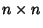Chessboard such that no two attack one another? The answer isqueens, which gives eight queens for the usual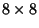board (Madachy 1979). The number of different ways thequeens can be placed on anchessboard so that no two queens may attack each other for the first feware 1, 0, 0, 2, 10, 4, 40, 92, ... (Sloane's A000170, Madachy 1979). The number of rotationally and reflectively distinct solutions are 1, 0, 0, 1, 2, 1, 6, 12, 46, 92, ... (Sloane's A002562; Dudeney 1970; p. 96). The 12 distinct solutions forare illustrated above, and the remaining 80 are generated by Rotation and Reflection (Madachy 1979).The minimum number of queens needed to occupy or attack all squares of anboard is 5. Dudeney (1970, pp. 95-96) gave the following results for the number of distinct arrangementsofqueens attacking or occupying every square of anboard for which every queen is attacked (protected'') by at least one other.Queens2 4 3 3 5 37 3 6 1 4 7 5

Dudeney (1970, pp. 95-96) also gave the following results for the number of distinct arrangementsofqueens attacking or occupying every square of anboard for which no two queens attack one another (they are not protected'').Queens1 2 1 1 3 1 3 4 2 3 5 2 4 6 17 4 7 1 5 8 91

Vardi (1991) generalizes the problem from a square chessboard to one with the topology of the Torus. The number of solutions forqueens withOdd are 1, 0, 10, 28, 0, 88, ... (Sloane's A007705). Vardi (1991) also considers the toroidal semiqueens'' problem, in which a semiqueen can move like a rook or bishop, but only on Positive broken diagonals. The number of solutions to this problem forqueens withOdd are 1, 3, 15, 133, 2025, 37851, ... (Sloane's A006717), and 0 for Even.

Chow and Velucchi give the solution to the question, How many different arrangements ofqueens are possible on an orderchessboard?'' as 1/8th of the Coefficient of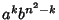in the Polynomial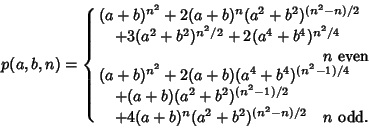Velucchi also considers the nondominating queens problem, which consists of placingqueens on an orderchessboard to leave a maximum numberof unattacked vacant cells. The first few values are 0, 0, 0, 1, 3, 5, 7, 11, 18, 22, 30, 36, 47, 56, 72, 82, ... (Sloane's A001366). The results can be generalized toqueens on anboard.

See also Bishops Problem, Chess, Kings Problem, Knights Problem, Knight's Tour, Rooks Problem

References

Ball, W. W. R. and Coxeter, H. S. M. Mathematical Recreations and Essays, 13th ed. New York: Dover, pp. 166-169, 1987.

Campbell, P. J. Gauss and the 8-Queens Problem: A Study in the Propagation of Historical Error.'' Historia Math. 4, 397-404, 1977.

Chow, T. and Velucchi, M. Different Dispositions in the Chessboard.'' http://www.cli.di.unipi.it/~velucchi/diff.txt.

Dudeney, H. E. The Eight Queens.'' §300 in Amusements in Mathematics. New York: Dover, p. 89, 1970.

Erbas, C. and Tanik, M. M. Generating Solutions to the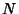-Queens Problem Using 2-Circulants.'' Math. Mag. 68, 343-356, 1995.

Erbas, C.; Tanik, M. M.; and Aliyzaicioglu, Z. Linear Congruence Equations for the Solutions of the-Queens Problem.'' Inform. Proc. Let. 41, 301-306, 1992.

Ginsburg, J. Gauss's Arithmetization of the Problem ofQueens.'' Scripta Math. 5, 63-66, 1939.

Guy, R. K. TheQueens Problem.'' §C18 in Unsolved Problems in Number Theory, 2nd ed. New York: Springer-Verlag, pp. 133-135, 1994.

Kraitchik, M. The Problem of the Queens'' and Domination of the Chessboard.'' §10.3 and 10.4 in Mathematical Recreations. New York: W. W. Norton, pp. 247-256, 1942.

Madachy, J. S. Madachy's Mathematical Recreations. New York: Dover, pp. 34-36, 1979.

Pólya, G. Über die doppelt-periodischen' Lösungen des-Damen-Problems.'' In Mathematische Unterhaltungen und Spiele (Ed. W. Ahrens). 1918.

Riven, I.; Vardi, I.; and Zimmerman, P. The-Queens Problem.'' Amer. Math. Monthly 101, 629-639, 1994.

Riven, I. and Zabih, R. An Algebraic Approach to Constraint Satisfaction Problems.'' In Proc. Eleventh Internat. Joint Conference on Artificial Intelligence, Vol. 1, August 20-25, 1989. Detroit, MI: IJCAII, pp. 284-289, 1989.

Ruskey, F. Information on theQueens Problem.'' http://sue.csc.uvic.ca/~cos/inf/misc/Queen.html.

Sloane, N. J. A. Sequences A001366, A000170/M1958, A006717/M3005, A007705/M4691, A002562/M0180 in An On-Line Version of the Encyclopedia of Integer Sequences.'' http://www.research.att.com/~njas/sequences/eisonline.html and extended entry in Sloane, N. J. A. and Plouffe, S. The Encyclopedia of Integer Sequences. San Diego: Academic Press, 1995.

Vardi, I. The-Queens Problems.'' Ch. 6 in Computational Recreations in Mathematica. Redwood City, CA: Addison-Wesley, pp. 107-125, 1991.

Velucchi, M. `Non-Dominating Queens Problem.'' http://www.cli.di.unipi.it/~velucchi/queens.txt.